STAT 330: 98-1

Assignment 5 Solutions

1. Chapter 6 Q 13: Let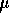be the population mean. Then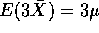so we must evaluate. The definition is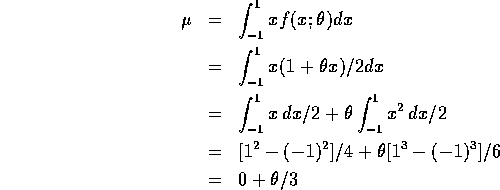so that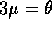.

2. Chapter 6 Q 15: The moment of order r is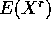which is given by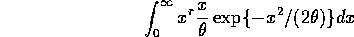Make the substitution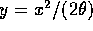for which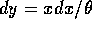and get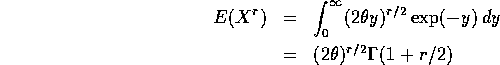For the special case r=2 we get the formula in the book (using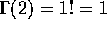).

1. According to the fact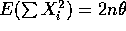so that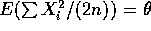. Thus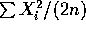is unbiased.
2. You square these numbers, add then divide by 20 and get 74.505 as in the appendix.

3. Chapter 6 Q 28:

1. The log of the density is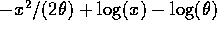so that the log likelihood is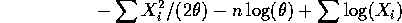To maximize this we take the derivative with respect toand set the result equal to 0 getting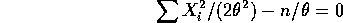which has root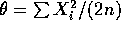which is the same as the unbiased estimate above.

2. The median, m, of the distribution satisfies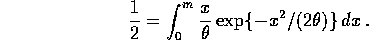The integral may be done by substitutingto get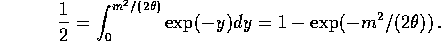Solve this to get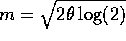and then the mle of m is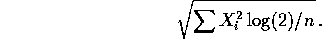4. Chapter 8 Q 26b: We are given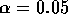so that the test rejects when t exceeds the t critical value on 7 degrees of freedom. The nearest curve in Table A.13 is for 6 degrees of freedom and the quantity d is (4.00-3.5)/1.25= .4 For 6 df this appears to correspond to a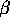of around 0.76 while for 9 df we would get about 0.65. My estimate is about a third of the way between them (because 7 is a third of the way between 6 and 9) or roughly .72.
5. Chapter 8 Q 30b: See the formula on page 319 and plug in to get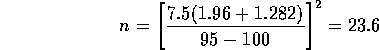so that n=24 would be needed. Notice that the formula for the sample size for a two sided level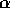test is just the same as that\ for a one sided level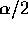test.

It is also acceptable to do this using the t-test graphs as in the previous question. In fact, I think this is what the text intended really. If so you get a sample size of 19.

6. Chapter 9 Q 6 b,c: The rejection region of the test is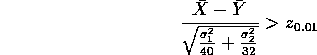which can be rewritten as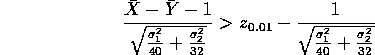The power function at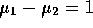is then the area to the right of the right hand side of this last formula under a standard normal curve. Plugging in numbers shows that we want the area to the left of -0.5 which is .31. That is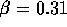approximately. To get a probability of type II error equal to 0.1 we need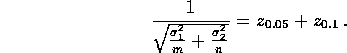Putting m=40 and plugging all the other numbers we get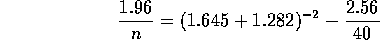which leads to n of roughly 37 (actually a bit over so rounding up to 38 would be normal).

7. Chapter 9 Q 12: This is just the formula at the foot of page 351. We get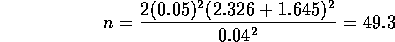which we round up to 50 for safety's sake.

8. Chapter 9 Q 73: This is a paired comparison problem. You take the differences, getting 3.2, -3.4, 0.4, 0.5, 0.3, -1.4, -0.3, 0, 3.5, -3.7, 3.7, 3.9, 1.6, 3.2. The one sample t statistic is 1.22 on 13 degrees of freedom leading to a 1 sided P value of 12% which is only weak evidence of higher TSI for the treatment than for the placebo.

Richard Lockhart
Fri Feb 13 15:37:53 PST 1998